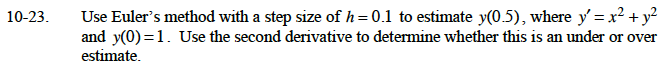### Home > CALC > Chapter Ch10 > Lesson 10.1.3 > Problem10-23

10-23.

Use Euler's method with a step size of h = 0.1 to estimate y(0.5), where y' = x2 + y2 and y(0) = 1. Use the second derivative to determine whether this is an under or over estimate. Homework Help ✎Since h = 0.1, this is equivalent to dx = 0.1.
Therefore dy = 0.1(x2 + y2).

Starting at (0, 1), dy = 0.1, so the next point is (0.1, 1.1).

Starting at (0.1, 1.1), dy = 0.122, so the next point is (0.2, 1.222).

Now repeat this process three more times.